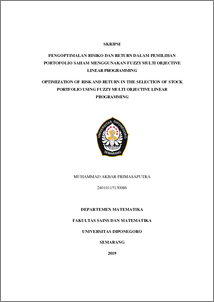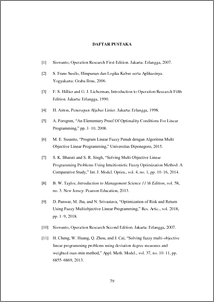# OPTIMIZATION OF RISK AND RETURN IN THE SELECTION OF STOCK PORTFOLIO USING FUZZY MULTI OBJECTIVE LINEAR PROGRAMMING

PRIMASAPUTRA, MUHAMMAD AKBAR (2019) OPTIMIZATION OF RISK AND RETURN IN THE SELECTION OF STOCK PORTFOLIO USING FUZZY MULTI OBJECTIVE LINEAR PROGRAMMING. Undergraduate thesis, UNDIP.Preview
PDF
195KbPDF
Restricted to Repository staff only

4062KbPreview
PDF
127Kb

## Abstract

The problem of stock selection in a stock portfolio is a challenge for investor. In this paper, several combined methods are used for portfolio selection, such as cluster analysis and analytical hierarchy process (AHP) to rank selected assets and fuzzy multi objective linear programming (FMOLP). Important factor of stock investment such as relative strength index (RSI), coefficient of variation (CV), earning yield (EY), and price to earning growth (PEG) ratio are also used in addition to the main factors of this problem; risk and return. Fuzzy multi objective linear programming is used to find the optimal value of the seven objective functions; risk, return, price to earning growth (PEG) ratio, relative strength index (RSI), coefficient of variation (CV), earning yield (EY), and AHP weighted score. FMOLP transforms the multi objective problems to a single objective by using a weighted adaptive approach based on the weight score that have been calculated using AHP. The results of the problem are then compared based on the lowest risk/return (CV) ratio. Keywords: stock portfolio selection, cluster analysis, analytic hierarchy proccess, fuzzy multi objective linear programming.

Item Type: Thesis (Undergraduate) Q Science > QA Mathematics Faculty of Science and Mathematics > Department of Mathematics 84266 INVALID USER 13 Jun 2022 11:01 13 Jun 2022 11:01

Repository Staff Only: item control page